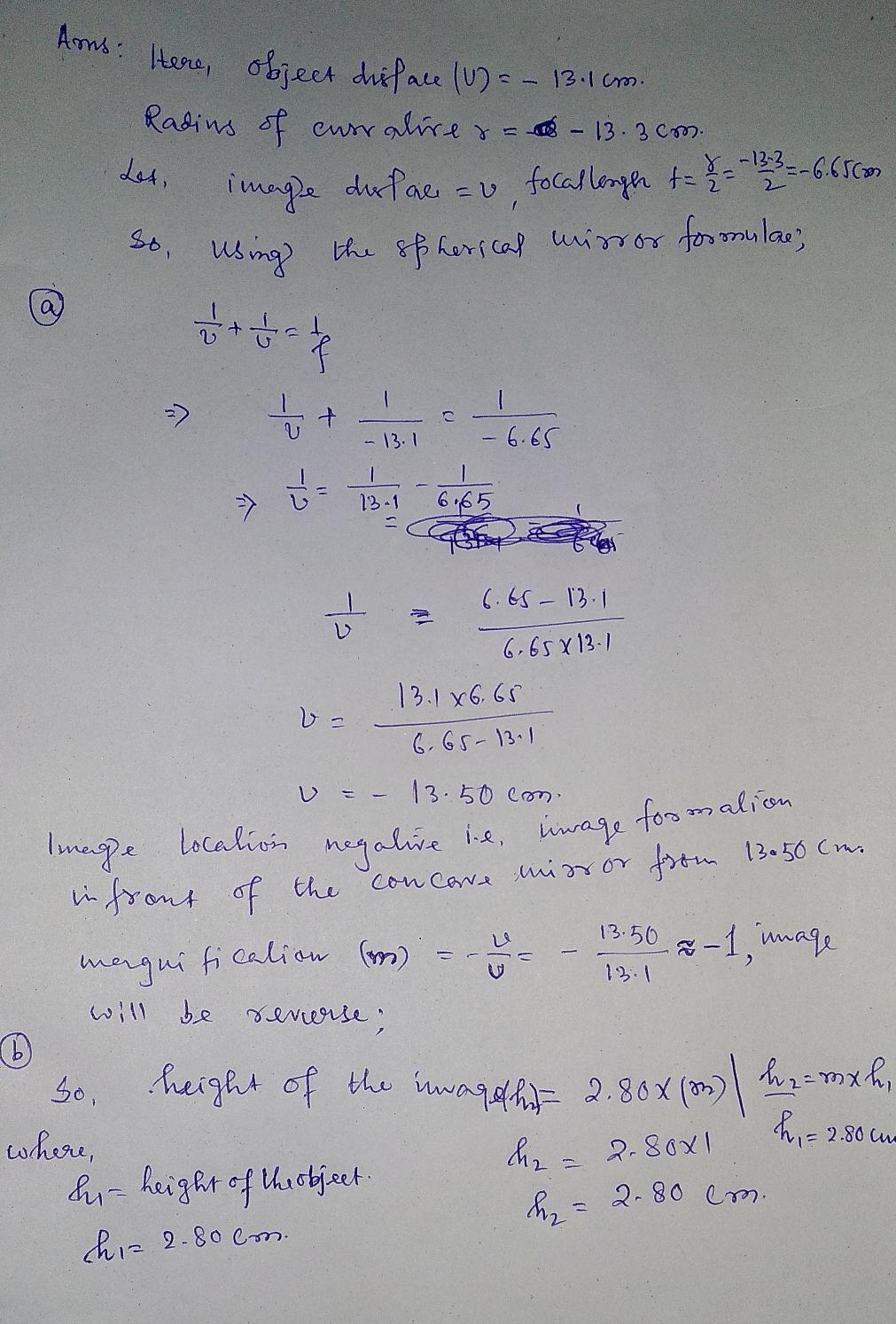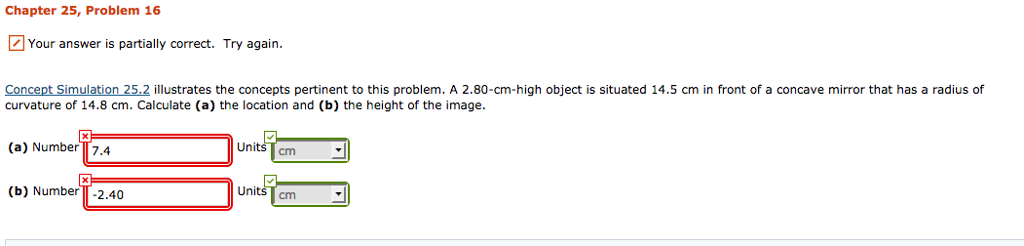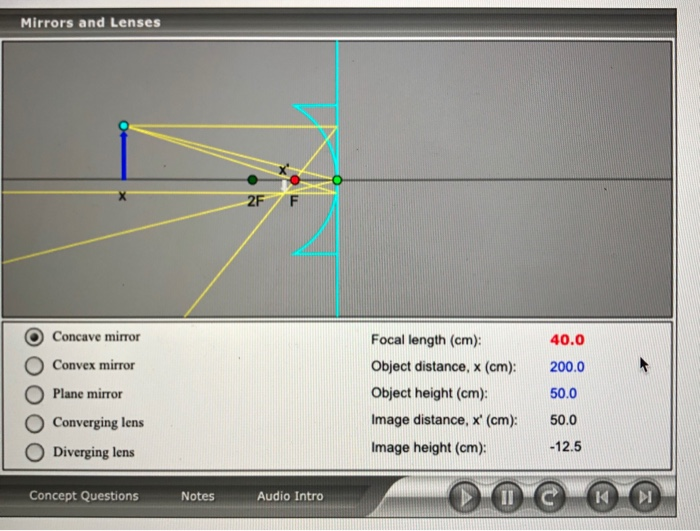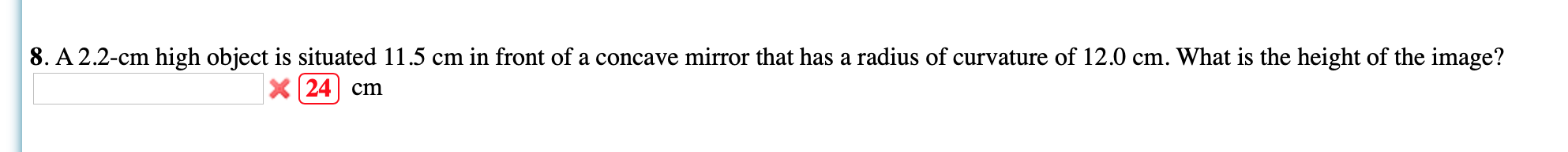Question

# illustrates the concepts pertinent to this problem. A 2.80-cm-high object is situated 13.1 cm in front...

illustrates the concepts pertinent to this problem. A 2.80-cm-high object is situated 13.1 cm in front of a concave mirror that has a radius of curvature of 13.3 cm. Calculate (a) the location and (b) the height of the image. (a) Number Units (b) Number Units#### Earn Coins

Coins can be redeemed for fabulous gifts.

Similar Homework Help Questions
• ### Concept Simulation 25.2 illustrates the concepts pertinent to this problem. A 1.60-cm-high object is situated 14.6...

Concept Simulation 25.2 illustrates the concepts pertinent to this problem. A 1.60-cm-high object is situated 14.6 cm in front of a concave mirror that has a radius of curvature of 9.46 cm. Calculate (a) the location and (b) the height of the image.

• ### Chapter 25, Problem 16 Your answer is partially correct. Try again. Concept Simulation 25.2 illustrates the...Chapter 25, Problem 16 Your answer is partially correct. Try again. Concept Simulation 25.2 illustrates the concepts pertinent to this problem. A 2.80-cm-high object is situated 14.5 cm in front of a concave mirror that has a radius of curvature of 14.8 cm. Calculate (a) the location and (b) the height of the image (a) Numberi74 (b) Number-2.40 Units cm Units cm

• ### A 2.30-cm-high object is situated 19.8 cm in front of a concave mirror that has a...

A 2.30-cm-high object is situated 19.8 cm in front of a concave mirror that has a radius of curvature of 12.4 cm. Calculate (a) the location and (b) the height of the image.

• ### A 2.4-cm high object is situated 15.0 cm in front of a concave mirror that has...

A 2.4-cm high object is situated 15.0 cm in front of a concave mirror that has a radius of curvature of 12.5 cm. What is the height of the image?

• ### 8. A 2.1-cm high object is situated 10.0 cm in front of a concave mirror that...

8. A 2.1-cm high object is situated 10.0 cm in front of a concave mirror that has a radius of curvature of 11.5 cm. What is the height of the image?

• ### A 5.0-cm-high object is situated 18.0 cm in front of a concave mirror that has a...

A 5.0-cm-high object is situated 18.0 cm in front of a concave mirror that has a radius of curvature of 11.0 cm. Use a ray diagram drawn to scale, measure the following. The mirror must be drawn to scale. a) the image distance (include the sign) (b) the height of the image

• ### A 5.0-cm-high object is situated 17.0 cm in front of a concave mirror that has a...

A 5.0-cm-high object is situated 17.0 cm in front of a concave mirror that has a radius of curvature of 10.0 cm. Use a ray diagram drawn to scale, measure the following. The mirror must be drawn to scale. (a) the image distance (include the sign) (b) the height of the image

• ### Mirrors and Lenses O O O O Concave mirror Convex mirror Plane mirror Converging lens Diverging...Mirrors and Lenses O O O O Concave mirror Convex mirror Plane mirror Converging lens Diverging lens Focal length (cm): Object distance, x (cm): Object height (cm): Image distance, x' (cm): Image height (cm): 40.0 200.0 50.0 50.0 -12.5 Concept Questions Notes Audio Intro Oo © Question 6 Concept Simulation 25.2 illustrates the concepts pertinent to this problem. A 2.10-cm-high object is situated 13.4 cm in front of a concave mirror that has a radius of curvature of 12.1 cm....

• ### 8. A 2.2-cm high object is situated 11.5 cm in front of a concave mirror that...8. A 2.2-cm high object is situated 11.5 cm in front of a concave mirror that has a radius of curvature of 12.0 cm. What is the height of the image? x 24 cm 3. A high energy pulsed laser emits 3.50 nano second-long pulse of average power 1.60 x 1011 W. The beam is cylindrical with 2.10 mm in radius. Determine the rms value of the B-field? x 3.478 T

• ### An object 3 cm high  is placed  40 cm in front of a concave spherical mirror with radius...

An object 3 cm high  is placed  40 cm in front of a concave spherical mirror with radius of curvature of 60 centimeters and an image of the object is observed. part a Make a drawing of the system part b Explain how you would calculate the focal length  and the position of the image. part c Calculate the height of the image. part d Indicate if the image is real or virtual and if it is upright or inverted.